# Electronics and Communication Engineering - Digital Electronics

26.

In following figure, the initial contents of the 4-bit serial in parallel out, right shift, shift register as shown in figure are 0110. After 3 clock pulses the contents of the shift register will be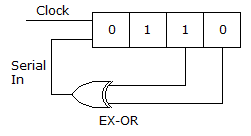A. 0000 B. 0101 C. 1010 D. 1110

Answer: Option C

Explanation: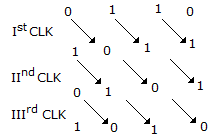27.

For the logic circuit of the given figure, the minimized expression is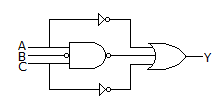A.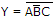B. Y = A + B + C C. Y = A + B D. Y = ABC

Answer: Option A

Explanation: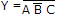+ A + C = A + B + C + A + C = A + C + B

=28.

Binary multiplication can be done by repeated addition.

 A. True B. False

Answer: Option A

Explanation:

No answer description available for this question. Let us discuss.

29.

Assertion (A): In totem pole output the output impedance is low.

Reason (R): TTL gate with active pull up should not be used in wired AND connection.

 A. Both A and R are correct and R is correct explanation of A B. Both A and R are correct but R is not correct explanation of A C. A is true, R is false D. A is false, R is true

Answer: Option B

Explanation:

No answer description available for this question. Let us discuss.

30.

A 4 bit synchronous counter uses flip flops with a delay time of 15 ns each. The time required for change of state is

 A. 15 ns B. 30 ns C. 45 ns D. 60 ns

Answer: Option A

Explanation:

In a synchronous counter clock input is applied to all flip flops simultaneously. Hence total delay time is 15 ns.

#### Current Affairs 2021

Interview Questions and Answers